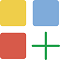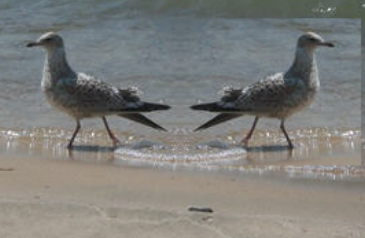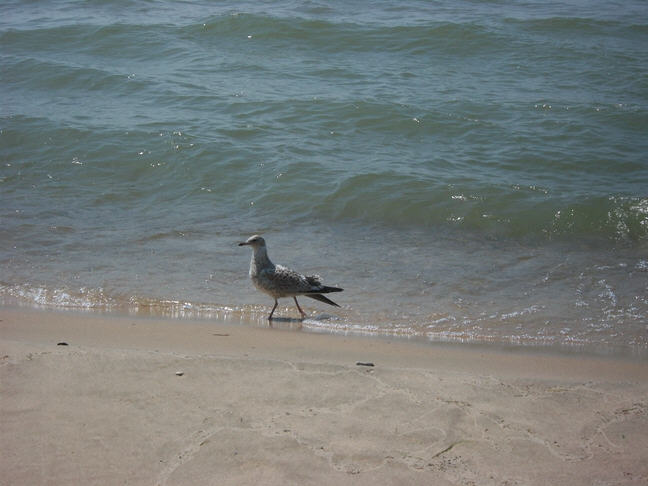Time estimate: 90 min.

# 8.9.5. Picture Lab A7: Mirroring Part of a Picture¶

Sometimes you only want to mirror part of a picture. For example, Figure 1 shows a temple in Greece that is missing a part of the roof called the pediment. You can use the Picture Explorer Repl to find the area that you want to mirror in temple.jpg to produce the picture on the right. If you do this you will find that you can mirror the rows from 27 to 96 (inclusive) and the columns from 13 to 275 (inclusive). You can change the starting and ending points for the row and column values to mirror just part of the picture.Figure 1: Greek temple before (left) and after (right) mirroring the pediment¶

To work with just part of a picture, change the starting and ending values for the nested for loops as shown in the following mirrorTemple method. This method also calculates the distance the current column is from the mirrorPoint and then adds that distance to the mirrorPoint to get the column to copy to.

public void mirrorTemple()
{
int mirrorPoint = 276;
Pixel leftPixel = null;
Pixel rightPixel = null;
int count = 0;
Pixel[][] pixels = this.getPixels2D();

// loop through the rows
for (int row = 27; row < 97; row++)
{
// loop from 13 to just before the mirror point
for (int col = 13; col < mirrorPoint; col++)
{
leftPixel = pixels[row][col];
rightPixel = pixels[row][mirrorPoint - col + mirrorPoint];
rightPixel.setColor(leftPixel.getColor());
}
}
}


You can test this with the mirrorTemple method below.

Picture Lab A7: Run to see mirrorTemple() working.

How many times was leftPixel = pixels[row][col]; executed? The formula for the number of times a nested loop executes is the number of times the outer loop executes multiplied by the number of times the inner loop executes. The outer loop is the one looping through the rows, because it is outside the other loop. The inner loop is the one looping through the columns, because it is inside the row loop.

How many times does the outer loop execute? The outer loop starts with row equal to 27 and ends when it reaches 97, so the last time through the loop row is 96. To calculate the number of times a loop executes, subtract the starting value from the ending value and add one. The outer loop executes 96 – 27 + 1 times, which equals 70 times. The inner loop starts with col equal to 13 and ends when it reaches 276, so, the last time through the loop, col will be 275. It executes 275 – 13 + 1 times, which equals 263 times. The total is 70 * 263, which equals 18,410.Coding Exercises

1. Check the calculation of the number of times the body of the nested loop executes by adding an integer count variable to the mirrorTemple method that starts out at 0 and increments inside the body of the loop. Print the value of count after the nested loop ends.

Picture Lab A7 Mirroring: Check the calculation of the number of times the body of the nested loop executes by adding an integer count variable to the mirrorTemple method that starts out at 0 and increments inside the body of the loop. Print the value of count after the nested loop ends which should be 18410.1. Write the method mirrorArms to mirror the arms on the snowperson (“snowperson.jpg”) to make a snowperson with 4 arms. Fork Picture Explorer Repl and change it to explore snowperson.jpg and find out the pixel coordinates to start and end the mirroring and the mirror point.

Picture Lab A7 Mirroring: Write the method mirrorArms to mirror the arms on the snowperson (“snowperson.jpg”) to make a snowperson with 4 arms.3. Write the method mirrorGull to mirror the seagull (“seagull.jpg”) to the right so that there are two seagulls on the beach near each other. Fork Picture Explorer Repl and change it to explore seagull.jpg and find out the pixel coordinates to start and end the mirroring and the mirror point.

Picture Lab A7 Mirroring: Write the method mirrorGull to mirror the seagull (“seagull.jpg”) to the right so that there are two seagulls on the beach near each other.

Images to use:

Data file: temple.jpgData file: snowperson.jpgData file: seagull.jpg